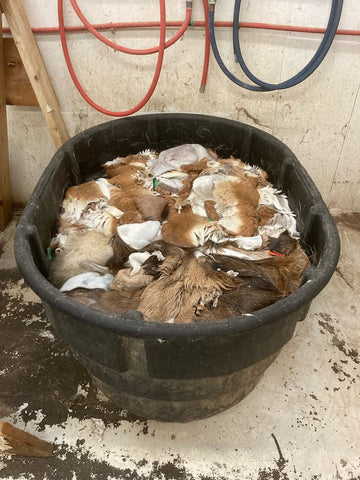# How to Determine a Float for Tanneries

When using our TanX AL-6 or TanX PR-3 tans, we recommend that the float be 350-375% of the weight of drained, shaved hides.  To determine the amount of water needed in gallons for a 350% float, use the following formula:

Hide weight times 350% = xx, then divide xx by 8.33 (which is the approx. weight of a gallon of water)

For example, 150 pounds of hides using a 350% float would be:

150 x 350% = 525 / 8.33 = 63 gallons

Or, you can change the % into a decimal:

150 x 3.5 = 525 / 8.33 = 63 gallons

When calculating a %, use the % key on your calculator.  We recommend rounding up to the nearest gallon.Notes:

• If using a static tan, use a float closer to 350%, you don’t want to pack the bath too tight, as the hides will need to have room to be agitated.
• For a wet drum tan, you may want to use a little tighter float.  You will know what % float works best with your particular wet drum process.
• We do not recommend running a float less than 330%.  Also, if you use a float greater than 400%, the tan will be too diluted.
• Shaving the hides prior to tanning will use less tan (less weight) and will also help the tan to penetrate better (being thinner).